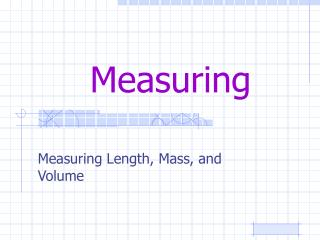DownloadDownload PresentationMeasuring

# Measuring

Télécharger la présentation## Measuring

- - - - - - - - - - - - - - - - - - - - - - - - - - - E N D - - - - - - - - - - - - - - - - - - - - - - - - - - -
##### Presentation Transcript

1. Measuring Measuring Length, Mass, and Volume

2. How long is the following? Ans. 7.7 cm

3. How long is the following? Ans. 13.1 cm or 13.17 cm How do we determine the second measurement?

4. How long is the following? Ans. 11.9 cm

5. How long is the following? Ans. 26.1 cm

6. How long is the following? Ans. 19.7 cm

7. How long is the following? Ans. 18.7 cm

8. How long is the following? Ans. 14.4 cm

9. How long is the following? Ans. 0.8 cm

10. What is the volume? Ans. 51 mL

11. What is the volume? Ans. 37 mL

12. What is the volume? Ans. 73 mL

13. What is the volume? Ans. 18.2 mL

14. What is the volume? Ans. 51 mL

15. What is the volume? Ans. 6.4 mL

16. What is the volume? Ans. 8.2 mL

17. What is the volume? Ans. 68 mL

18. What is the mass? Ans. 164.8 g

19. What is the mass? Ans. 324.4 g

20. What is the mass? Ans. 45.7

21. What is the mass? Ans. 2.9

22. What is wrong with me?